$$\require{color}$$

### Continuous Combinatorics

The study of limit objects of growing combinatorial structures.

This talk: graph limits $${\color{orange}\mathcal{G}} = (G_i)_{i=1}^\infty,$$ where $G_i$ is a graph on $i$ vertices.

### Graphons (Graph-functions)

###### [Lovász, Szegedy, 2006]

Limits of adjacency matrices: symmetric measurable functions $$W\colon[0,1]^2\to[0,1]$$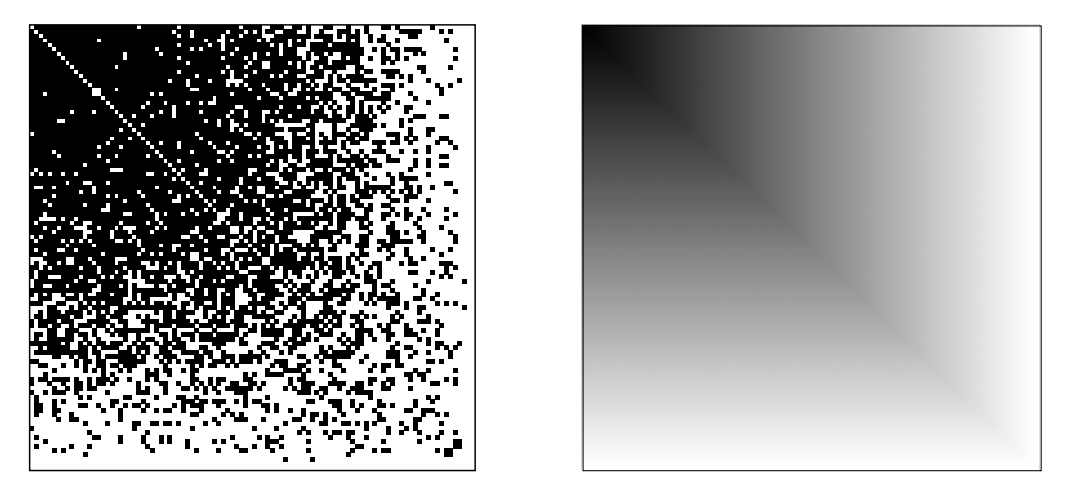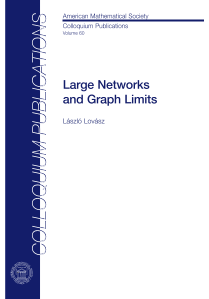### Flag Algebras

###### [Razborov, 2007]

Record only the limits of subgraph densities: $$\phi(H) = \lim_{i\to\infty} (\text{density of H in G_i}).$$ Flag Algebras consider the algebraic relations between different values $\phi(H)$ and $\phi(H')$.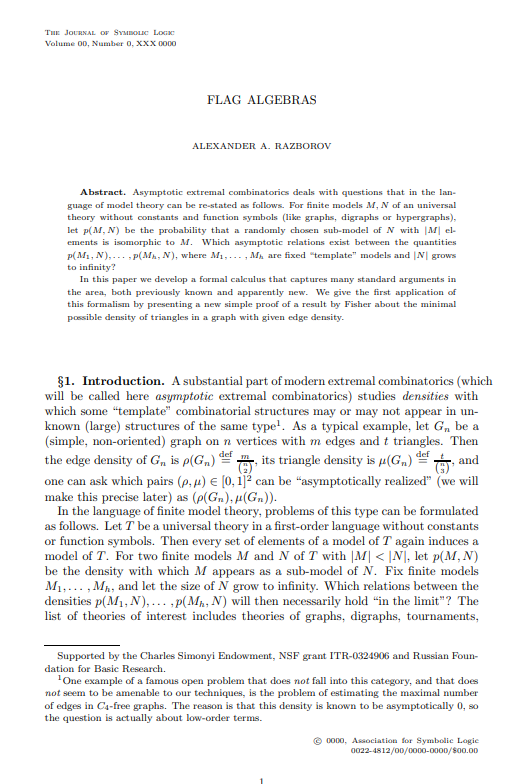### Graphons and Flag Algebras

Flag algebras' limit functionals $$\phi\colon \{\text{finite graphs}\}\to[0,1]$$ correspond to graphons "up to isomorphism".

They form a compact metric space.

### Subgraph densities

The density of a graph $\color{green}H$ in $\color{orange}\mathcal{G}= (G_i)_{i=1}^\infty$ is $$\phi({\color{green}H}) := \lim_{i\to\infty} \mathbb{P}[{\color{red}\sigma_i}({\color{green}H}) \text{ is a subgraph of }{\color{orange}G_i}],$$ where ${\color{red}\sigma_i}$ is a random permutation in $S_i$.

### Triangle free graphs

Maximize the edge density in a triangle free sequence $\mathcal{G}$ of graphs of increasing size:We saw thatbut how can we prove an upper bound?

### Multiplying subgraph densities

To multiply two subgraph densities, we glue together the graphs:These relationships are independent of $\mathcal{G}$, motivating the definitionwhere a graph $H$ now stands for the function $$H(\mathcal{G}) = \phi_{\mathcal{G}}(H).$$

We can fix entries of the $\sigma_i$ to fix (flag) some vertices, and extend the gluing operation to partially labeled graphs (Flags):### Flag Sums-of-Squares

• Flags $F$ send graph sequences to real numbers: $$F (\mathcal{G}) \in [0,1]$$
• Then so do real linear combinations of flags
• Squares of real numbers are nonnegative:We can average Flags over all choices of labels, unlabeling them:We can now find an upper bound for the edge density in triangle free graphs:As with polynomial optimization, we can model Flag-SOS using semidefinite programming.

### The space of graph limits

We saw that triangle free graphs have at most edge density $\frac{1}{2}$.

What happens if we allow some triangles?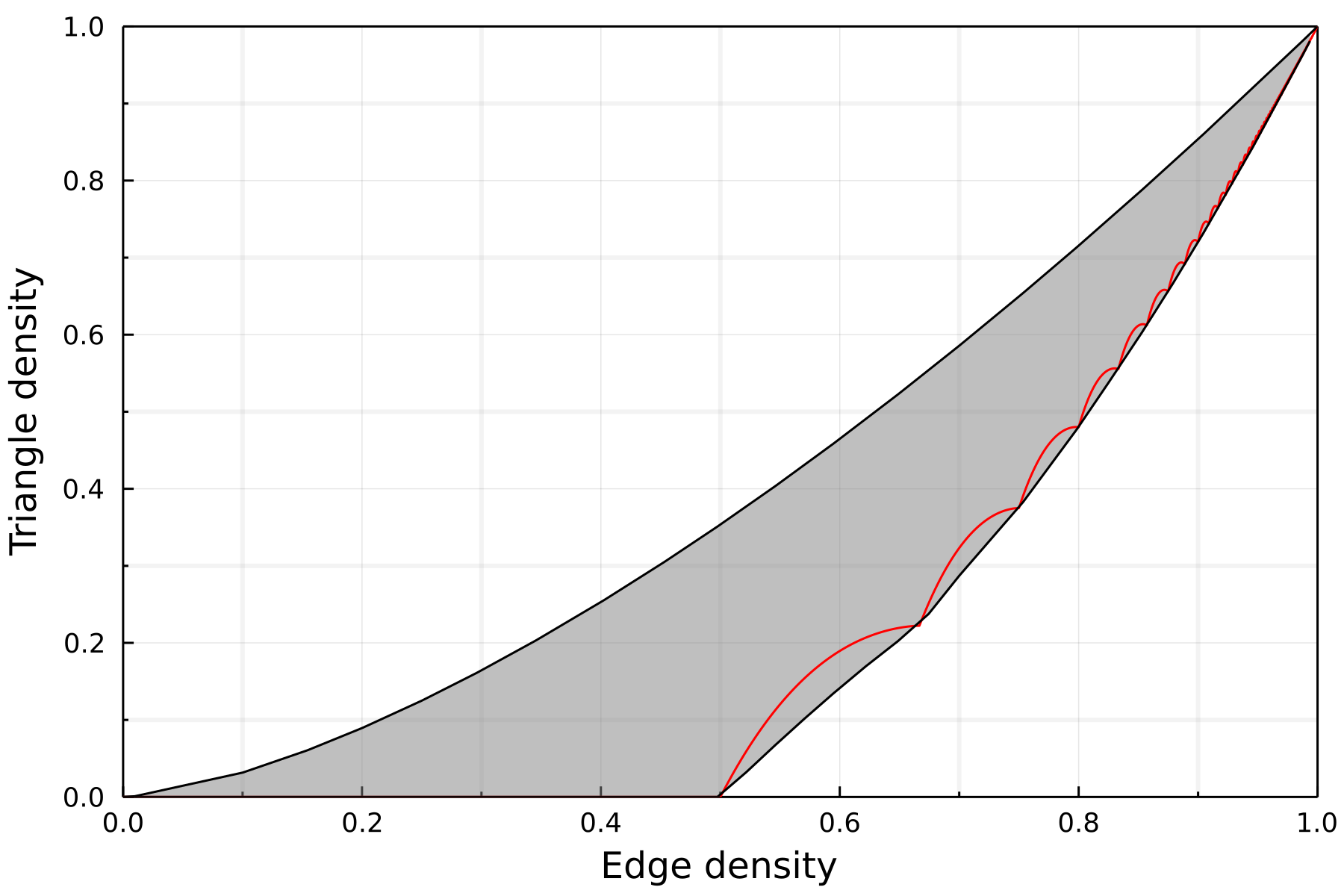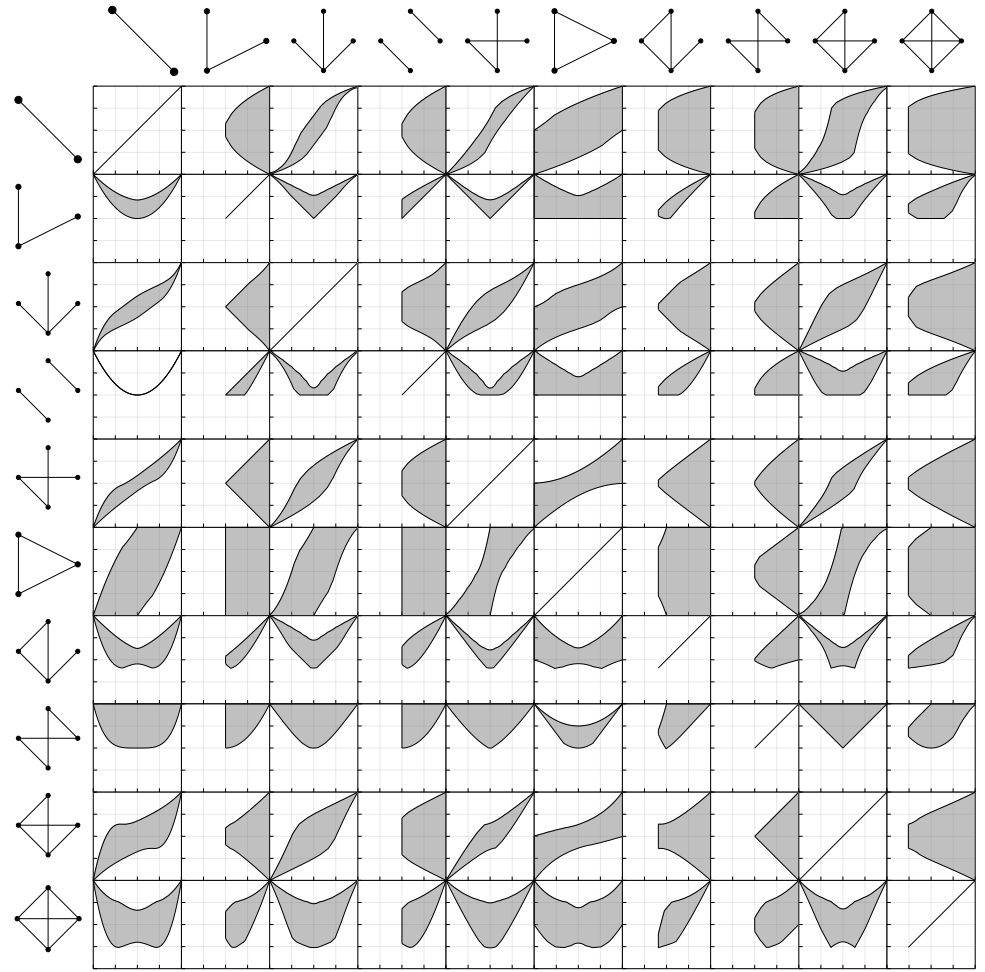• Linear combinations of subgraph densities are continuous functions in this space.
• How can we move in the space without leaving it?
• Can we define directional derivatives of flags?

### Derivatives of flags

Razborov introduces two derivatives:

• In direction of deleting a vertex $v$.
• In direction of deleting an edge $e$.

Lovász translates the definition to graphons.

Diao, Guillot, Khare, Bajaratnam consider Gateaux derivatives in the space of graphons.

### $\partial_v$: Deleting the vertex ${\color{orange}v}$

###### (We assume all flags with label ${\color{orange}v}$ converge)### $\partial_v$: Deleting the vertex ${\color{orange}v}$

###### (We assume all flags with label ${\color{orange}v}$ converge)### $\partial_v$: Deleting the vertex ${\color{orange}v}$

###### (We assume all flags with label ${\color{orange}v}$ converge)### $\partial_e$: Deleting the edge ${\color{orange}e}$### $\partial_e$: Deleting the edge ${\color{orange}e}$### Using the derivatives in optimization

Let ${\color{orange}f}$ be a linear combination of subgraph densities, with minimizer $\mathcal{G}$ .

• Then $\partial_{\color{red}v} {\color{orange}f}(\mathcal{G}) = 0$ for nearly all vertices ${\color{red}v}$.
• Then $\partial_{\color{red}e} {\color{orange}f}(\mathcal{G}) \geq 0$ for nearly all edges ${\color{red}e}$.

### Using the derivatives in optimization

\begin{align} \min_{\mathcal{G}}\enspace& {\color{orange}f}(\mathcal{G}) &= \min_{\mathcal{G}}\enspace& {\color{orange}f}(\mathcal{G})\\ &&\text{s.t. }& \mathbb{P}[\partial_{\color{red}v} {\color{orange}f}(\mathcal{G}) = 0]=1, \\ &&& \mathbb{P}[\partial_{\color{red}e} {\color{orange}f}(\mathcal{G}) \geq 0]=1. \end{align}

We can find better SOS certificates in the quadratic module given by these constraints.

### Proof idea

• Assume there is an $\varepsilon$-subset of vertices/edges that violates the constraints.
• Show that there exists a subset of $\Theta(n)$ vertices resp. $\Theta(n^2)$ edges we can remove consecutively to shift the objective.

Problem: We have to pic the elements "independently enough".

### Main contribution

We show that the proofs generalize to more complicated actions on three or more vertices.

Main observation: We only ever need to apply the operation $\Theta(n^2)$ times! We can construct bigger "independent" sets probabilistically.

### Sidorenko's Conjecture

For fixed edge density $\rho$, the Erdős–Rényi random graph $\mathrm{ER}(\rho, n)$ minimizes the density of every bipartite graph as $n\to\infty$.

For every bipartite graph $H$,### Progress on Sidorenko's Conjecture

• [Mulholland, Smith 1959]: paths

### Progress on Sidorenko's Conjecture

• [Mulholland, Smith 1959]: paths
• [Sidorenko 1991]: trees, even cycles, $K_{n,m}$, bipartite graphs with at most $4$ vertices on one side

### Progress on Sidorenko's Conjecture

• [Mulholland, Smith 1959]: paths
• [Sidorenko 1991]: trees, even cycles, $K_{n,m}$, bipartite graphs with at most $4$ vertices on one side
• [Hitomi 2008]: Hypercube graphs, norming graphs

### Progress on Sidorenko's Conjecture

• [Sidorenko 1991]: trees, even cycles, $K_{n,m}$, bipartite graphs with at most $4$ vertices on one side
• [Hitomi 2008]: Hypercube graphs, norming graphs
• [Conlon, Fox, Sudakov 2010]: If one vertex connects to all vertices on the other side

### Progress on Sidorenko's Conjecture

• [Hitomi 2008]: Hypercube graphs, norming graphs
• [Conlon, Fox, Sudakov 2010]: If one vertex connects to all vertices on the other side
• [Sidorenko 1991], [Li, Szegedy 2011], [Kim, Lee, Lee 2013]: Various recursive constructions

### Progress on Sidorenko's Conjecture

• [Conlon, Fox, Sudakov 2010]: If one vertex connects to all vertices on the other side
• [Sidorenko 1991], [Li, Szegedy 2011], [Kim, Lee, Lee 2013]: Various recursive constructions
• [Conlon, Lee 2018]: For every bipartite graph $H$ exists a $p$ such that the $p$-blow up of $H$ is Sidorenko.

### Progress on Sidorenko's Conjecture

• [Sidorenko 1991], [Li, Szegedy 2011], [Kim, Lee, Lee 2013]: Various recursive constructions
• [Conlon, Lee 2018]: For every bipartite graph $H$ exists a $p$ such that the $p$-blow up of $H$ is Sidorenko.
• [Blekherman, Raymond, Singh, Thomas 2018]: $P_3-e^3$ is not (rational) flag-SOS. The smallest open case $K_{5,5}\setminus C_{10}-e^{15}$ is not not (rational) flag-SOS.

### Progress on Sidorenko's Conjecture

• [Blekherman, Raymond, Singh, Thomas 2018]: $P_3-e^3$ is not (rational) flag-SOS. The smallest open case $K_{5,5}\setminus C_{10}-e^{15}$ is not not (rational) flag-SOS.
• [Garg, Raymond, Redlich 2022]: Many cases of Sidorenko's Conjecture cannot be shown using flag-SOS. Most known cases can potentially be recovered using flag-SOS.
Interest for alternative proofs for $P_3-e^3\geq 0$!

### Hinge-switch derivative

We define the derivative $\partial_{\text{hinge}}$ based on the operation on vertices $i,j,k$, which swaps the edges $\{i,j\}$ and $\{i,k\}$.

### SOS based proof of a small case of Sidorenko's

We found an exact flag-SOS certificate for \begin{align} 0 = \min\enspace& P_3 - e^3\\ \text{s.t. }& \mathbb{P}[\partial_{\text{hinge}}(P_3 - e^3) \geq 0 ]=1 \end{align}

## Software:

A Julia package implementing multiple symmetry reduced flag-SOS hierarchies for arbitrary Flags will be available soon.

## Paper:

Very much work in progress (Open for co-authors!). Other work related to flag algebras is in my thesis.